## 前言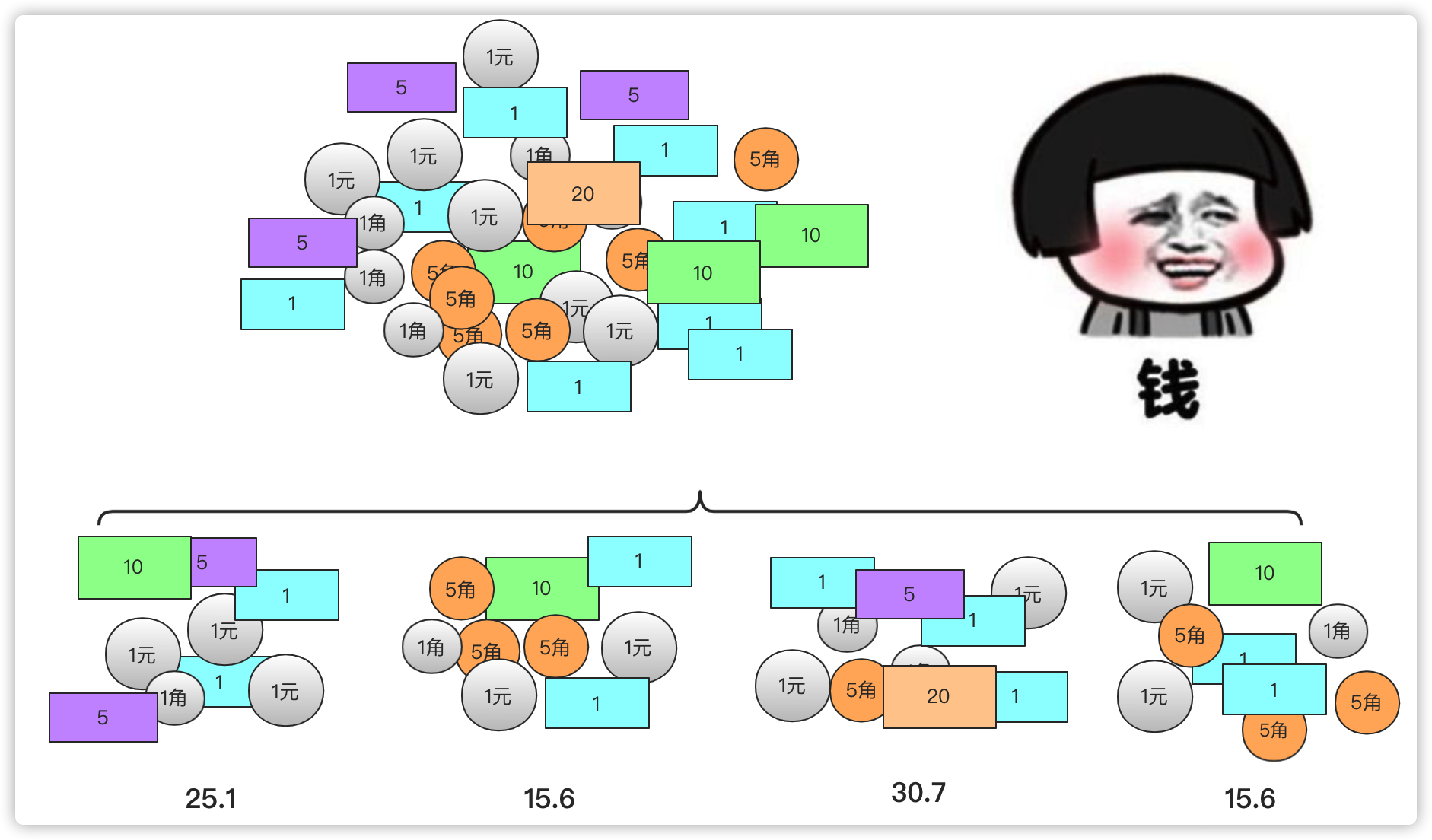• 计算每个小堆钱的方式和计算最大堆钱的方式是相同的(区别在于体量上)
• 然后大堆钱总和其实就是小堆钱结果之和。这样其实就有一种分治的思想。## 分治算法介绍

• 分(Divide)：递归解决较小的问题(到终止层或者可以解决的时候停下)
• 治(Conquer)：递归求解，如果问题够小直接求解。
• 合并(Combine)：将子问题的解构建父类问题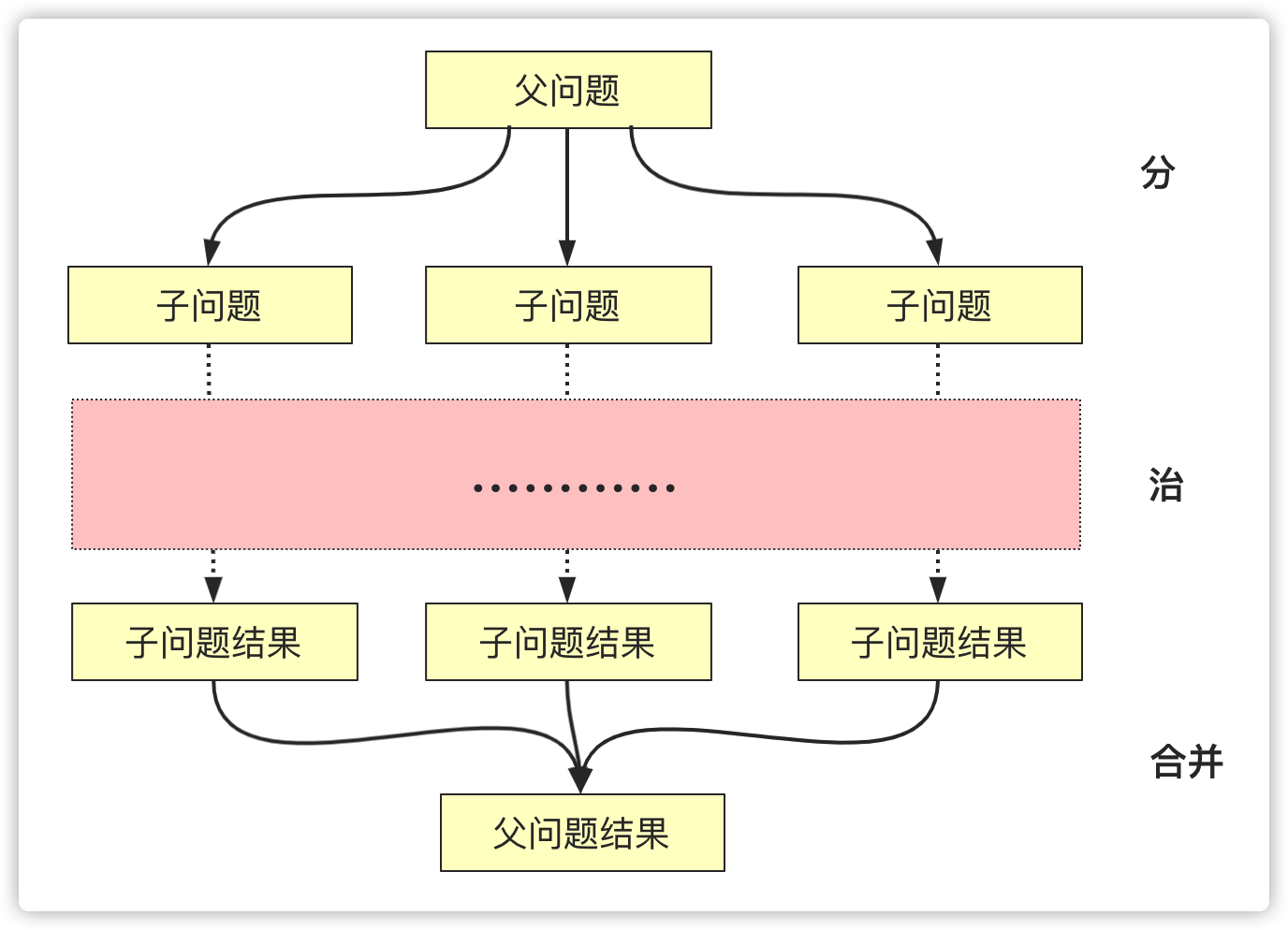1 . 原问题规模通常比较大，不易直接解决，但问题缩小到一定程度就能较容易的解决。

2 . 问题可以分解为若干规模较小、求解方式相同(似)的子问题。且子问题之间求解是独立的互不影响。

3 . 合并问题分解的子问题可以得到问题的解。

## 分治算法经典问题

1 . 子问题完全独立就是原问题的答案可完全由子问题的结果推出。

2 . 子问题不完全独立，有些区间类的问题或者跨区间问题使用分治可能结果跨区间,在考虑问题的时候需要仔细借鉴下。

### 二分搜索

• 序列有序
• 结果为一个值

``````public int searchInsert(int[] nums, int target) {
if(nums>=target)return 0;//剪枝
if(nums[nums.length-1]==target)return nums.length-1;//剪枝
if(nums[nums.length-1]<target)return nums.length;
int left=0,right=nums.length-1;
while (left<right) {
int mid=(left+right)/2;
if(nums[mid]==target)
return mid;
else if (nums[mid]>target) {
right=mid;
}
else {
left=mid+1;
}
}
return left;
}``````

### 快速排序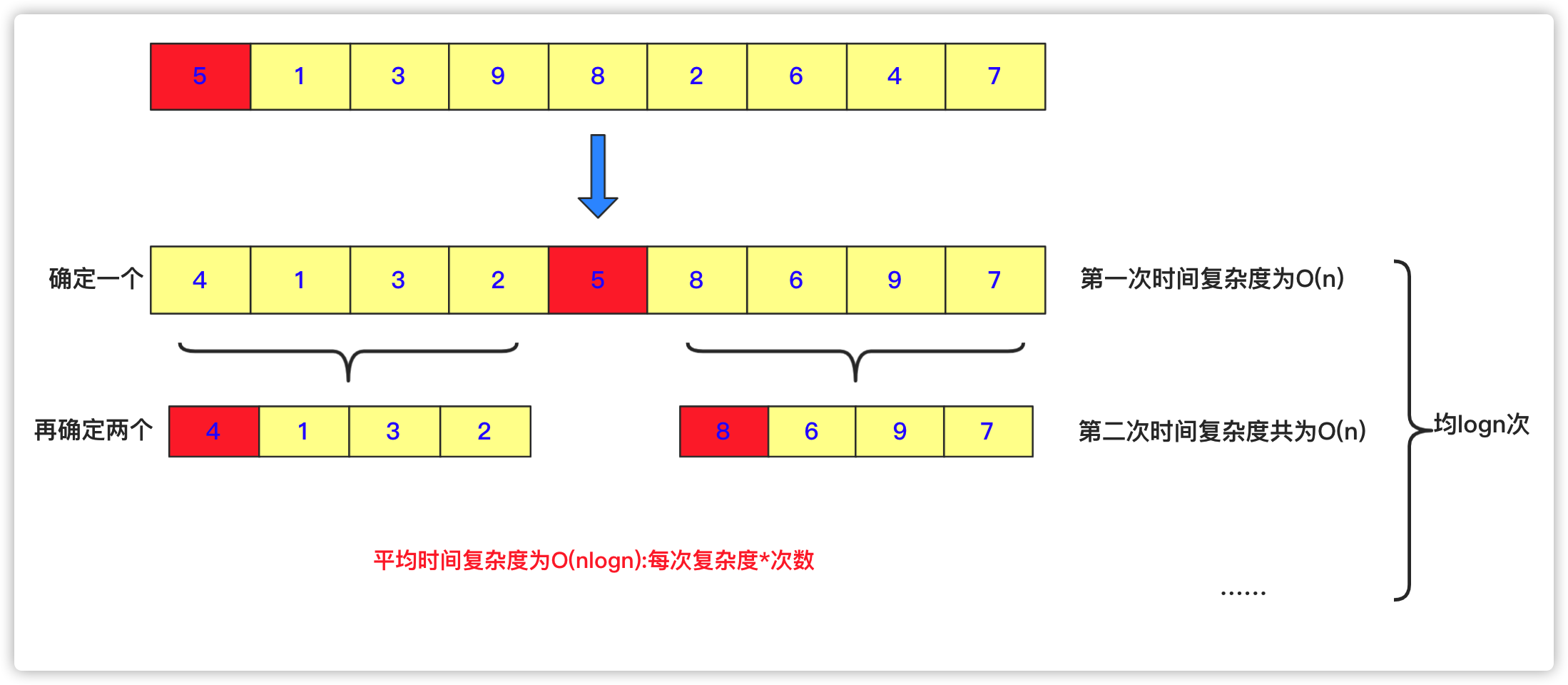``````public void quicksort(int [] a,int left,int right)
{
int low=left;
int high=right;
//下面两句的顺序一定不能混，否则会产生数组越界！！！very important！！！
if(low>high)//作为判断是否截止条件
return;
int k=a[low];//额外空间k，取最左侧的一个作为衡量，最后要求左侧都比它小，右侧都比它大。
while(low<high)//这一轮要求把左侧小于a[low],右侧大于a[low]。
{
while(low<high&&a[high]>=k)//右侧找到第一个小于k的停止
{
high--;
}
//这样就找到第一个比它小的了
a[low]=a[high];//放到low位置
while(low<high&&a[low]<=k)//在low往右找到第一个大于k的，放到右侧a[high]位置
{
low++;
}
a[high]=a[low];
}
a[low]=k;//赋值然后左右递归分治求之
quicksort(a, left, low-1);
quicksort(a, low+1, right);
}
``````

### 归并排序(逆序数)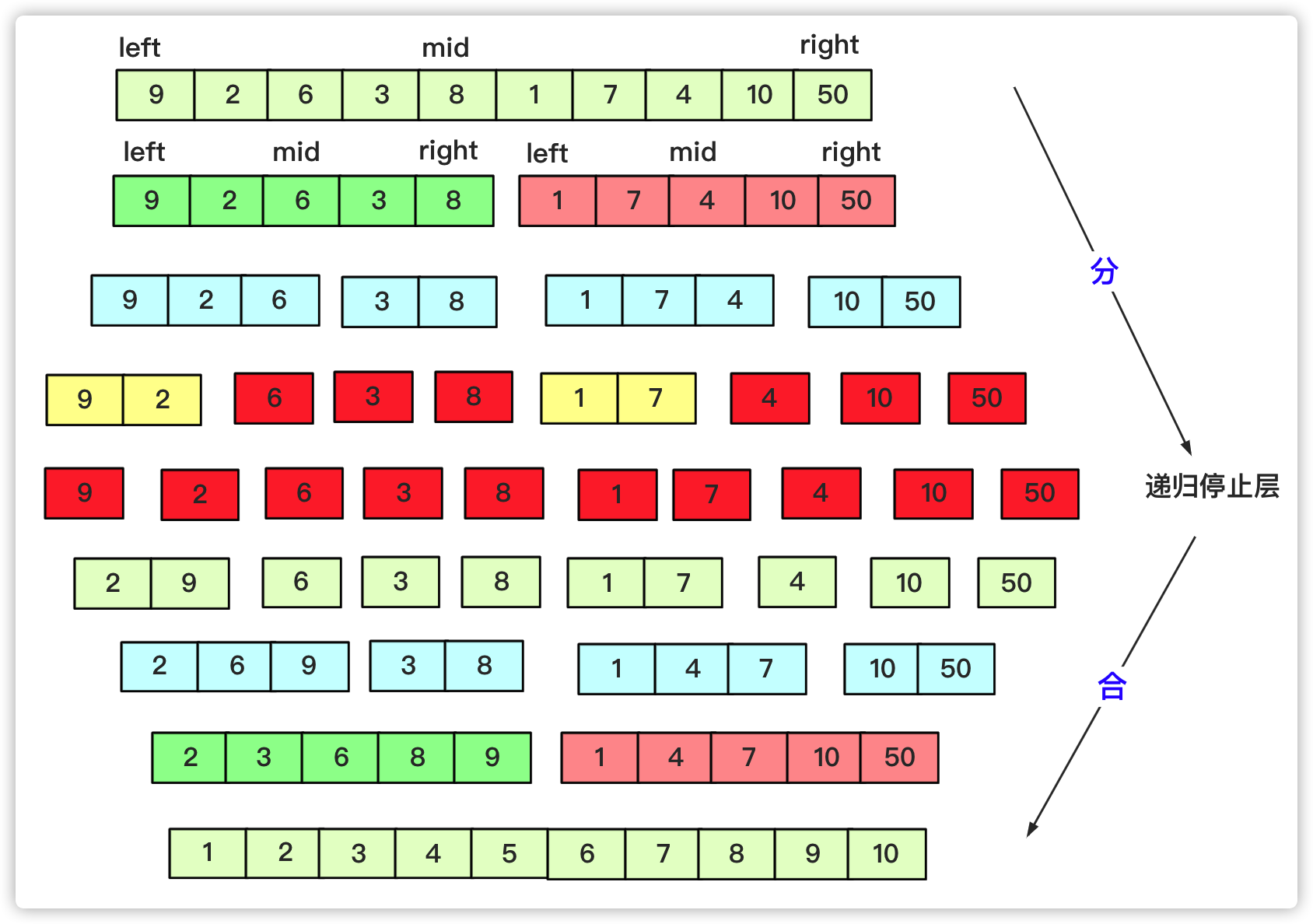``````private static void mergesort(int[] array, int left, int right) {
int mid=(left+right)/2;
if(left<right)
{
mergesort(array, left, mid);
mergesort(array, mid+1, right);
merge(array, left,mid, right);
}
}

private static void merge(int[] array, int l, int mid, int r) {
int lindex=l;int rindex=mid+1;
int team[]=new int[r-l+1];
int teamindex=0;
while (lindex<=mid&&rindex<=r) {//先左右比较合并
if(array[lindex]<=array[rindex])
{
team[teamindex++]=array[lindex++];
}
else {
team[teamindex++]=array[rindex++];
}
}
while(lindex<=mid)//当一个越界后剩余按序列添加即可
{
team[teamindex++]=array[lindex++];

}
while(rindex<=r)
{
team[teamindex++]=array[rindex++];
}
for(int i=0;i<teamindex;i++)
{
array[l+i]=team[i];
}
}``````

### 最大子序列和

• 完全在中间的左侧
• 完全在中间的右侧
• 包含中间左右两个节点的一个序列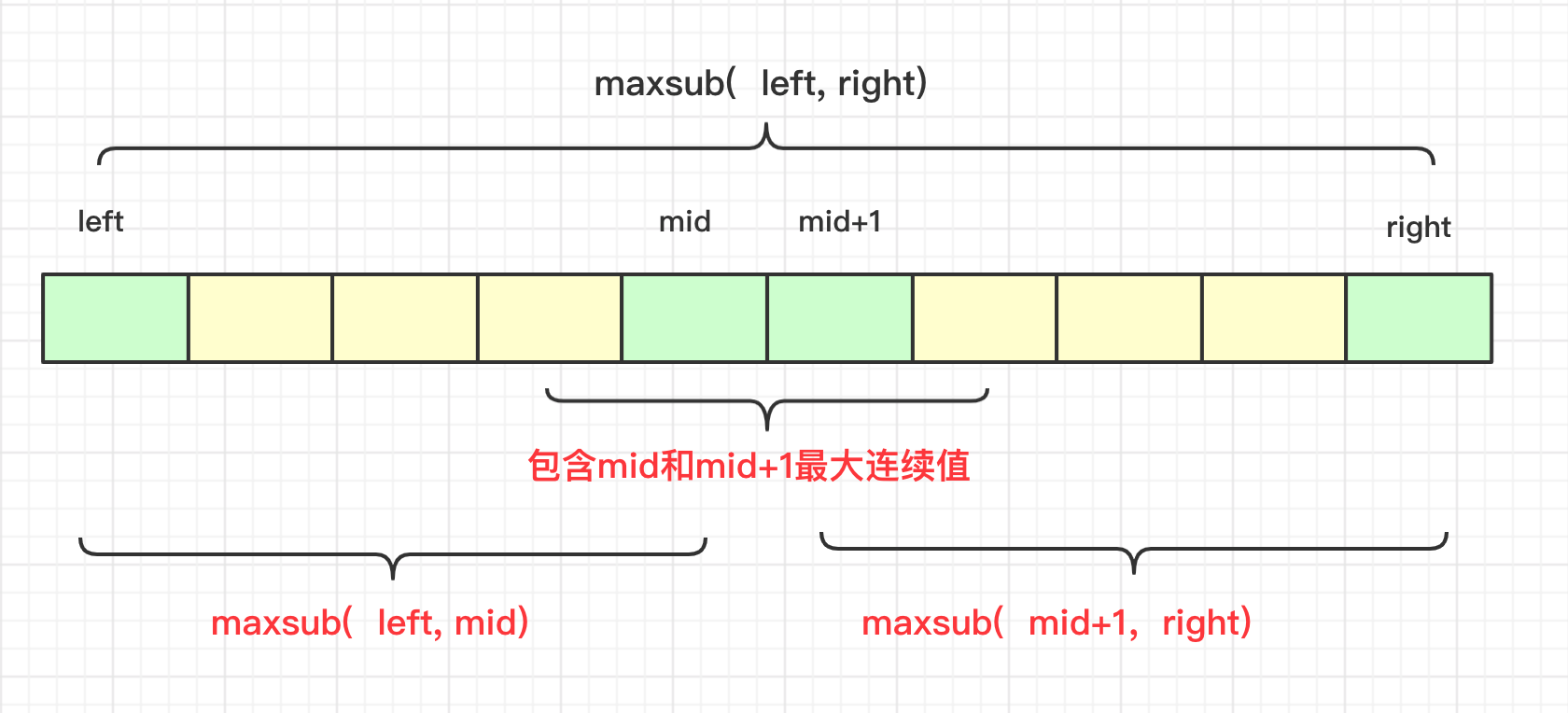``````public int maxSubArray(int[] nums) {
int max=maxsub(nums,0,nums.length-1);
return max;
}
int maxsub(int nums[],int left,int right)
{
if(left==right)
return  nums[left];
int mid=(left+right)/2;
int leftmax=maxsub(nums,left,mid);//左侧最大
int rightmax=maxsub(nums,mid+1,right);//右侧最大

int midleft=nums[mid];//中间往左
int midright=nums[mid+1];//中间往右
int team=0;
for(int i=mid;i>=left;i--)
{
team+=nums[i];
if(team>midleft)
midleft=team;
}
team=0;
for(int i=mid+1;i<=right;i++)
{
team+=nums[i];
if(team>midright)
midright=team;
}
int max=midleft+midright;//中间的最大值
if(max<leftmax)
max=leftmax;
if(max<rightmax)
max=rightmax;
return  max;
}``````

### 最近点对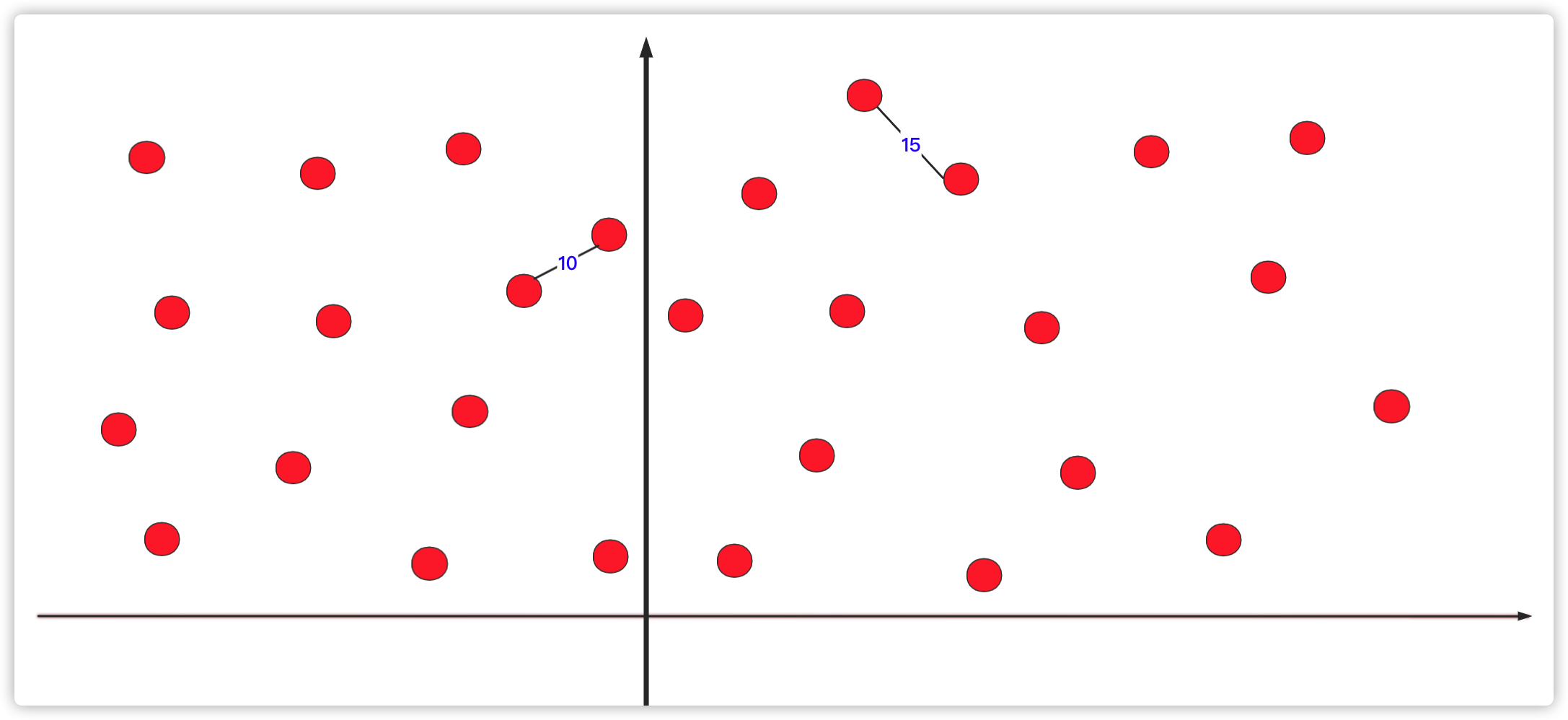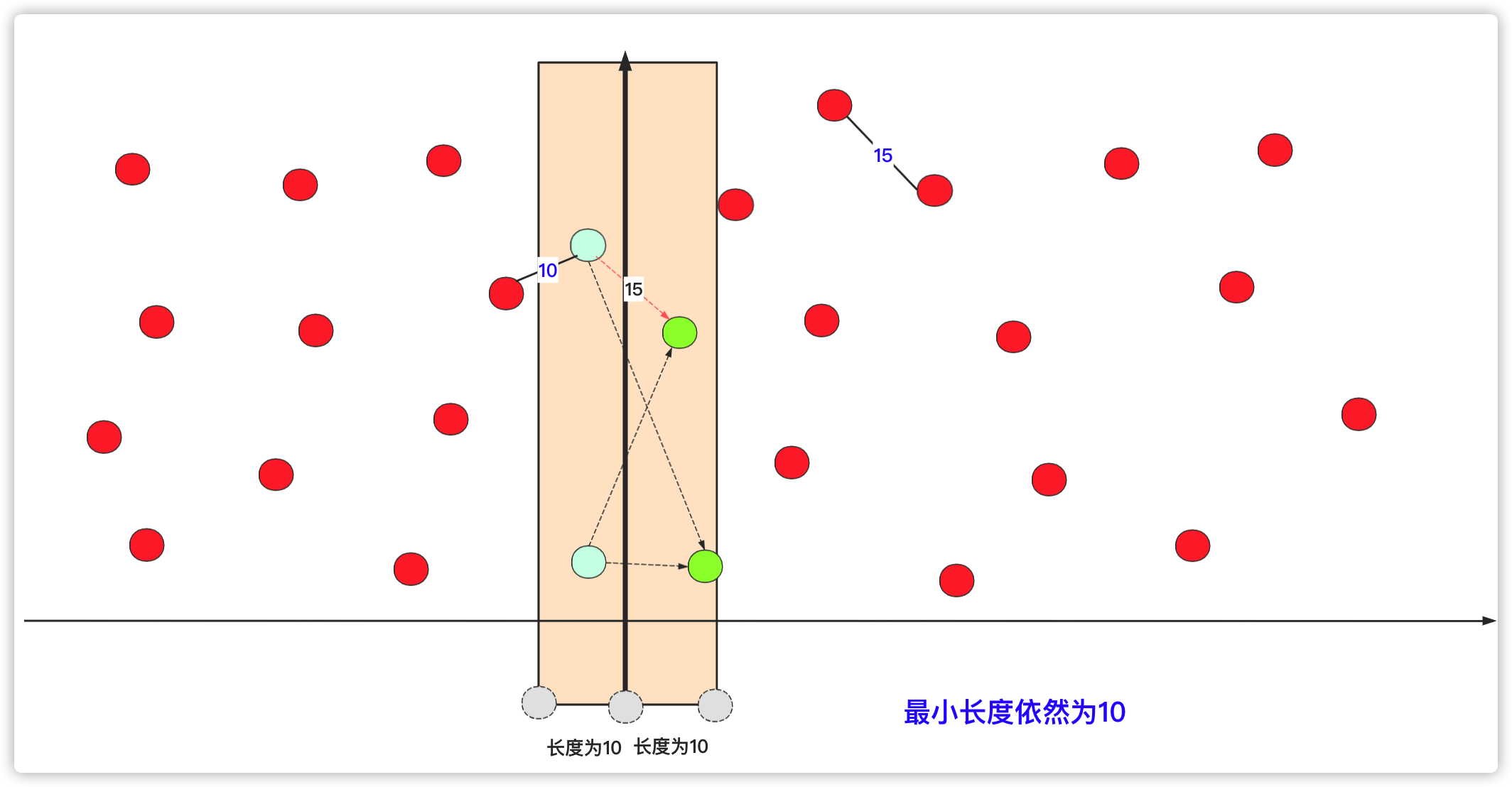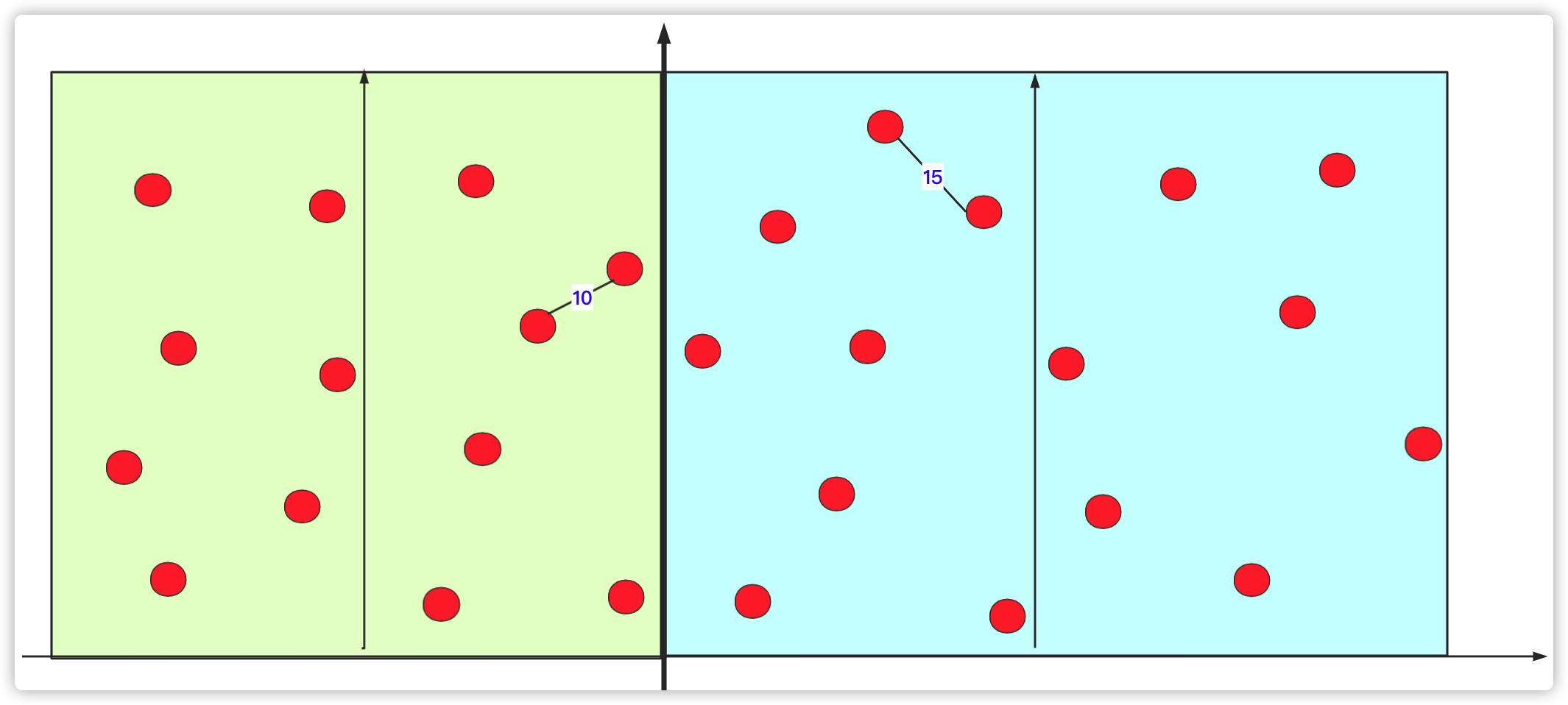``````import java.io.BufferedReader;
import java.io.IOException;
import java.io.OutputStreamWriter;
import java.io.PrintWriter;
import java.io.StreamTokenizer;
import java.util.ArrayList;
import java.util.Arrays;
import java.util.Comparator;
import java.util.List;
public class Main {
static int n;
public static void main(String[] args) throws IOException {
PrintWriter out = new PrintWriter(new OutputStreamWriter(System.out));
//List<node>list=new ArrayList();
while(in.nextToken()!=StreamTokenizer.TT_EOF)
{
n=(int)in.nval;if(n==0) {break;}
node no[]=new node[n];

for(int i=0;i<n;i++)
{
in.nextToken();double x=in.nval;
in.nextToken();double y=in.nval;
no[i]=new node(x,y);
}
Arrays.sort(no, com);
double min= search(no,0,n-1);
out.println(String.format("%.2f", Math.sqrt(min)/2));out.flush();
}
}
private static double search(node[] no, int left,int right) {
int mid=(right+left)/2;
double minleng=0;
if(left==right) {return Double.MAX_VALUE;}
else if(left+1==right) {minleng= (no[left].x-no[right].x)*(no[left].x-no[right].x)+(no[left].y-no[right].y)*(no[left].y-no[right].y);}
else minleng= min(search(no,left,mid),search(no,mid,right));
int ll=mid;int rr=mid+1;
while(no[mid].y-no[ll].y<=Math.sqrt(minleng)/2&&ll-1>=left) {ll--;}
while(no[rr].y-no[mid].y<=Math.sqrt(minleng)/2&&rr+1<=right) {rr++;}
for(int i=ll;i<rr;i++)
{
for(int j=i+1;j<rr+1;j++)
{
double team=0;
if(Math.abs((no[i].x-no[j].x)*(no[i].x-no[j].x))>minleng) {continue;}
else
{
team=(no[i].x-no[j].x)*(no[i].x-no[j].x)+(no[i].y-no[j].y)*(no[i].y-no[j].y);
if(team<minleng)minleng=team;
}
}
}
return minleng;

}
private static double min(double a, double b) {
// TODO 自动生成的方法存根
return a<b?a:b;
}
static Comparator<node>com=new Comparator<node>() {

@Override
public int compare(node a1, node a2) {
// TODO 自动生成的方法存根
return a1.y-a2.y>0?1:-1;
}};
static class node
{
double x;
double y;
public node(double x,double y)
{
this.x=x;
this.y=y;
}
}
}
``````

## 结语

1. 点赞在看， 您的肯定是我在平台创作的源源动力。

2. 微信搜索「bigsai」，关注我的公众号，不仅免费送你电子书，我还会第一时间在公众号分享知识技术。加我还可拉你进力扣打卡群一起打卡LeetCode。